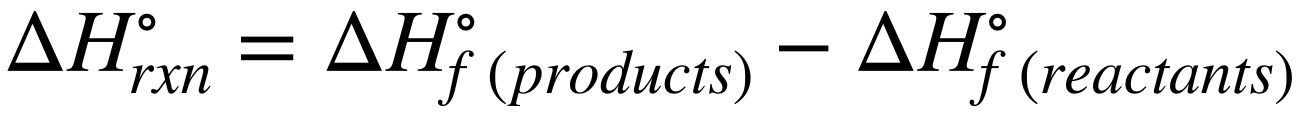# Problem: Acetylene burns in air according to the following equation:C2H2(g) + 5/2O2(g) ⟶ 2CO2(g) + H2O(g)          ΔH°rxn = −1255.8 kJGiven ΔH°f of CO2(g) = −393.5 kJ/mol and ΔH°f of H2O(g) = −241.8 kJ/mol, find ΔH°f of C2H2(g).

###### FREE Expert Solution

Recall: The enthalpy of reaction (∆H˚rxn) can be calculated from the reactants' and products' enthalpy of formation (∆H˚f) using the equation:90% (75 ratings)###### Problem Details

Acetylene burns in air according to the following equation:

C2H2(g) + 5/2O2(g) ⟶ 2CO2(g) + H2O(g)          ΔH°rxn = −1255.8 kJ

Given ΔH°f of CO2(g) = −393.5 kJ/mol and ΔH°f of H2O(g) = −241.8 kJ/mol, find ΔH°f of C2H2(g).

Frequently Asked Questions

What scientific concept do you need to know in order to solve this problem?

Our tutors have indicated that to solve this problem you will need to apply the Enthalpy of Formation concept. You can view video lessons to learn Enthalpy of Formation. Or if you need more Enthalpy of Formation practice, you can also practice Enthalpy of Formation practice problems.

What professor is this problem relevant for?

Based on our data, we think this problem is relevant for Professor Ocando's class at QC CUNY .

What textbook is this problem found in?

Our data indicates that this problem or a close variation was asked in Chemistry: The Molecular Nature of Matter and Change - Silberberg 8th Edition. You can also practice Chemistry: The Molecular Nature of Matter and Change - Silberberg 8th Edition practice problems.